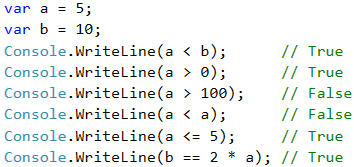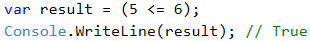# Comparing Numbers

In programming, we can compare values using the following operators:

• Operator < (less than)
• Operator > (greater than)
• Operator <= (less than or equals)
• Operator >= (greater than or equals)
• Operator == (equals)
• Operator != (different from)

When compared, the result is a Boolean value true or false, depending on whether the result of the comparison is true or false.

## Video: Comparing Numbers

Watch the video lesson about comparing numbers: https://youtu.be/KTdqDWg7Wf8.

## Examples for Comparing NumbersNote that when printing the true and false values in C # language, they are printed with a capital letter, respectively True and False.

## Comparison Operators

In C#, we can use the following comparison operators:

Operator Notation Applicable for
Equals == numbers, strings, dates
Not equal !=
Greater than > numbers, dates, other comparable objects
Greater than or equals >=
Less than <
Less than or equals <=

The following example demonstrates how to use comparison operators in expressions: